• Index
•
• Previous
• Next
•
• Search

# 5.2   Methods

• MacSlope provides five methods of limit equilibrium analysis to calculate the factor of safety: Ordinary, Bishop's Simplified, Janbu's Simplified, Spencer's, and General Limit Equilibrium.
• All methods are based on limit equilibrium formulations for calculating the factor of safety. This means they are based on the equations of static equilibrium, and the factor of safety is defined as the factor by which the available shear strength (ie. resisting forces) must be reduced to be in a state of just-stable limiting equilibrium with the shear stress (ie. driving forces)
• This section is not intended to provided a detailed review of the theory and formulation of the available methods, but rather a brief overview of the problem and the key differences between methods. The user's attention is directed to some of the published works from the References section for more detailed information on the methods.
• Set the analysis method and associated parameters by selecting Analysis Settingsin the tool palette, or selecting Show Analysis Settings under the Analysis Menu.

## Problem Definition

• The figure below shows a typical compound slip with a piezometric surface, tension crack, and ponded water. The dimension and force variables used in the various methods are defined in the figure. The definition of an external line load, although not modeled in this problem, is shown for reference in "grey. MacSlope's force and dimension variables are based upon Figure 1 from Fredlund, D.G, and Krahn, J., 1977. Comparison of slope stability methods of analysis. Canadian Geotechnical Journal. Vol. 39. pp. 665-683.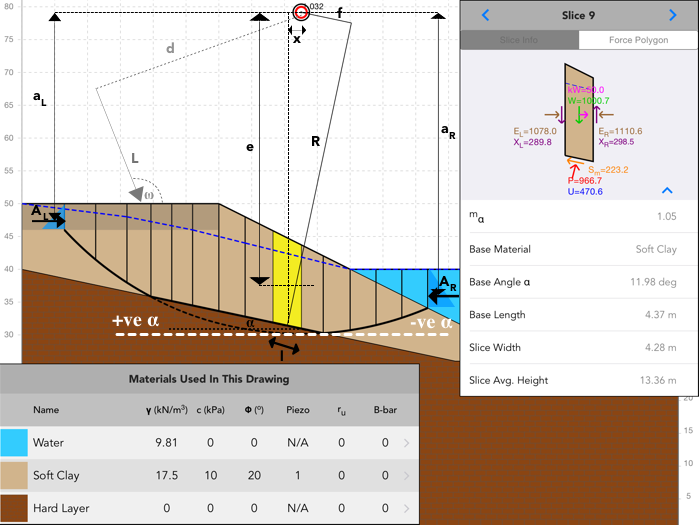Definition of Dimension and Force Variables

## Factor of Safety Equations

• Methods satisfying moment equilibrium use the following moment factor of safety equation: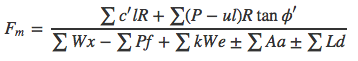• Methods satisfying force equilibrium use the following force factor of safety equation: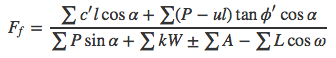• All methods with the exception of the ordinary method use the following equation for the normal force at the slice base: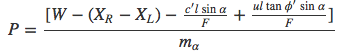Where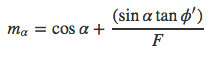## Ordinary Method

• Satisifies moment equilibrium.
• Neglects all interslice forces.
• Calculates factor of safety directly (no iterations).
• Not accurate for non-circular slip surfaces, and effective stress analyses with pore pressures. Therefore it is not recommended.
• Uses following equation for the normal force at the slice base: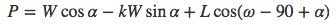## Bishop's Simplified

• Satisifies moment equilibrium.
• Includes interslice normal forces but neglects interslice shear forces.
• Factor of safety calculated iteratively until within the specified Convergence Tolerance.
• If the factor of safety does not converge within number of attempts defined in Iteration Limit, it is declared non-convergent.

## Janbu's Simplified

• Satisifies force equilibrium.
• Includes interslice normal forces but neglects interslice shear forces.
• Factor of safety calculated iteratively until within the specified Convergence Tolerance.
• If factor of safety does not converge within number of attempts defined in Iteration Limit, it is declared non-convergent.
• An empirical correction factor can be applied to try to account for the effect of interslice shear forces. This generally raises the calculated factor of safety by up to 10%.

## Spencer's Method

• Satisifies force and moment equilibrium.
• Includes interslice normal and shear forces, with a constant relationship between them. (X / E = tanθ or X = E * λ in GLE terms)
• Moment and force factor of safety are calculated iteratively for varying values of θ until moment and force factor of safety are within the specified Convergence Tolerance.
• If factor of safety does not converge within number of attempts defined in Iteration Limit, it is declared non-convergent.

## General Limit Equilibrium

• Satisifies force and moment equilibrium.
• Includes interslice normal and shear forces, with a specified interslice force function relationship between them. (X = E * f(x) * λ in GLE terms)
• Specified interslice force functions available are: Constant and Half-sine.
• Moment and force factor of safety are calculated over the specified range of λ at the specified interval, and the intersection of the two curves is the reported factor of safety.
• Once an analysis is complete, use the Inspector Toolto plot the Moment-Force Factor of Safety, which gives a complete picture of how the moment and force factors of safety vary over the range of λ.